# DAV Class 3 Maths Chapter 10 Brain Teasers Solutions

The DAV Maths Book Class 3 Solutions and DAV Class 3 Maths Chapter 10 Brain Teasers Solutions of Capacity offer comprehensive answers to textbook questions.

## DAV Class 3 Maths Ch 10 Brain Teasers Solutions

Question 1.

(a) Standard unit of length is—
(i) km
(ii) m
(iii) cm
(iv) 1
Solution:
(ii) m

(b) 10 l is equal to—
(i) 100 ml
(ii) 10 ml
(iii) 10000 ml
(iv) 1000 ml
Solution:
(iii) 10000 ml(c) Approximate wt of an apple is—
(i) 3 kg
(ii) 2 kg
(iii) 150 g
(iv) 500 g
Solution:
(iii) 150 g

(d) 5 m can be the length of a—
(i) pen
(ii) saree
(iv) book
Solution:
(ii) saree

(e) A ribbon is measured in—
(i) m
(ii) 1
(iii) g
(iv) kg
Solution:
(i) mQuestion 2.
Write the most appropriate unit to measure the following.

(a) Length of a blackboard. _____
Solution:
metres

(b) Weight of a mobile phone. _____
Solution:
Gram

(c) Distance between the earth and the moon. _____
Solution:
Kilometre

(d) Volume of orange juice from 2 oranges. _____
Solution:
millilitres

(e) Quantity of rice needed for a family of three for a weak. _____
Solution:
kilogram

(f) Length of a sarce. _____
Solution:
Metres

(g) Weight of a iron box. _____
Solution:
kilogram

(h) Quantity of milk in a feeding bottle. _____
Solution:
litres.Question 3.
Fill in the blanks.

(a) 7 kg = _____ g
Solution:
7000

(b) 11 m = _____ cm
Solution:
1100

(c) 5 l = _____ ml
Solution:
5000

(d) 10 kg = _____ g
Solution:
10000

(e) 9 m = _____ cm
Solution:
900Question 4.
The length of a cloth is 1 m 12 cm more than its width. If the width is 1 m 50 cm, find the length of the cloth.
Solution:
Width is 1 m 50 cm and length is 1 m 12 cm more than width so to find length we have to add the extra quantity in width.
width = 1 m 50 cm
length is more than width = 1 m 12 cm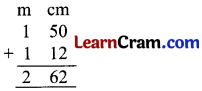Length = 2 m 62 cm
2 m 62 cm is length of cloth.

Question 5.
A fruitseller purchased 19 kg 750 g apples and 25 kg 525 g mangoes. If he sells 11 kg 600 g apples and 23 kg 345 g mangoes, find the wt of fruits left to be sold.
Solution:
Quantity of apples purchased = 19 kg 750 g
Quantity of mangoes purchased = 25 kg 525 gTotal fruits purchased = 45 kg 275 g

Quantity of apples solds = 11 kg 600 g
Quantity of mangoes sold = 23 kg 345 g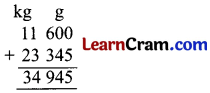Total sold = 34 kg 945 g

weight of fruits left to be sold = 45 kg 275 g – 34 kg 945 g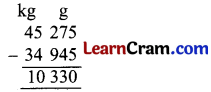10 kg 330 g fruits are left to be sold.Question 6.
Riya bought 5 kg of almonds. She gave 800 g to her maid and 1 kg 300 g to her neighbour. How much almonds are left with Riya?
Solution:
Almonds given to maid = 0 kg 800 g
Almonds given to neighbours = 1 kg 300 g
= 2 kg 100 gTotal almond purchased = 5 kg 000
Given to maid & neighbours = 2 kg 100 g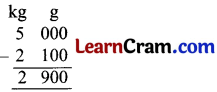Almonds Left = 2 kg 900 g

Question 7.
Sheelu wants to prepare a cold drink for herself. She pours 200 ml of cold water, 75 ml of rose syrup and 300 ml of milk in a glass. What is the quantity of cold drink Sheelu has made?
Solution:
Cold water added = 200 ml
Rose syrup added = 75 ml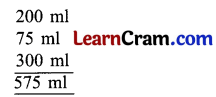575 ml of cold drink is made by Sheelu.Question 8.

(a) 17 kg 158 g, 11 kg 342 g and 14 kg 250 g
Solution:
42 kg 750 g

(b) 7 m 35 cm, 21 m 46 cm and 3 m 15 cm
Solution:
31 m 96 cm

Question 9.
Subtract:

(a) 9 l 350 ml from 17 l 736 ml
Solution: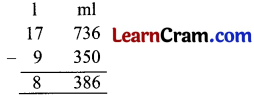= 8 l 386 ml

(b) 76 m 25 cm from 100 m 75 cm
Solution:= 24 m 50 cmQuestion 1.
(i) 13 l 685 ml, 31 l 120 ml and 20 l 100 ml
Solution:= 64 l 905 ml

(ii) 11 l 210 ml, 10 l 420 ml and 16 l 310 ml
Solution:= 37 l 940 mlQuestion 2.

(a) 61 l 475 ml from 89 l 586 ml
Solution: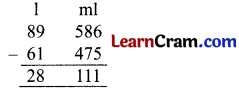= 28 l 111 ml
(b) 36 l 675 ml – 26 l 439 ml
Solution:= 10 l 236 ml

(c) 9 l 439 ml from 12 l 178 ml
Solution:= 2 l 739 mlQuestion 3.
Which unit of measurement will you choose to express the following?

(a) wt of apple.
Solution:
kilogram

(b) Ink in a pen.
Solution:
millilitre

(c) Roohafza in glass of water/milk.
Solution:
millilitre

(d) Height of boy.
Solution:
centimetre

(e) wt of brick.
Solution:
kilogram.Question 4.
Fill in the blanks:

(a) 80 l = _____ ml.
Solution:
80000 ml

(b) 9 l 350 ml = _____ ml.
Solution:
9350 ml

(c) 5 Litres 145 millilitres = _____ ml.
Solution:
5145 ml

(d) 3 l 200 ml = _____ ml.
Solution:
3200 ml

(e) 8 l 750 ml = _____ ml.
Solution:
8750 ml.Question 5.
A basket contained 40 kg 650 g of fruits. Out of which 20 kg 210 g are pears and 11 kg 100 g are grapes and rest are oranges. Find the weight of oranges.
Solution:
The weight of Pears = 20 kg 210 g
The weight of grapes = 11 kg 100 gTotal wt of fruits in basket = 40 kg 650 g
wt of pears + grapes = 31 kg 310 g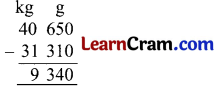wt of oranges = 9 kg 340 g.